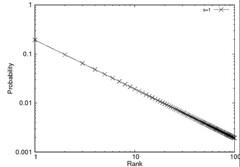# Zipf Distribution Random Generator in Java

When I carry out some experiments, I usually make synthetic data sets generated by  some probability distributions.  Especially, Zipf distribution is frequently used for a synthetic data set. Zipf distribution is  one of the discrete power law probability distributions. You can get detail information from Zipf’s law in Wikipedia. Anyway, I attached my own java class for zip distribution. Below graphs are generated by my own java code and the gnuplot.

```import java.util.Random;

public class ZipfGenerator {
private Random rnd = new Random(System.currentTimeMillis());
private int size;
private double skew;
private double bottom = 0;

public ZipfGenerator(int size, double skew) {
this.size = size;
this.skew = skew;

for(int i=1;i < size; i++) {
this.bottom += (1/Math.pow(i, this.skew));
}
}

// the next() method returns an random rank id.
// The frequency of returned rank ids are follows Zipf distribution.
public int next() {
int rank;
double friquency = 0;
double dice;

rank = rnd.nextInt(size);
friquency = (1.0d / Math.pow(rank, this.skew)) / this.bottom;
dice = rnd.nextDouble();

while(!(dice &lt; friquency)) {
rank = rnd.nextInt(size);
friquency = (1.0d / Math.pow(rank, this.skew)) / this.bottom;
dice = rnd.nextDouble();
}

return rank;
}

// This method returns a probability that the given rank occurs.
public double getProbability(int rank) {
return (1.0d / Math.pow(rank, this.skew)) / this.bottom;
}

public static void main(String[] args) {
if(args.length != 2) {
System.out.println("usage: ./zipf size skew");
System.exit(-1);
}

ZipfGenerator zipf = new ZipfGenerator(Integer.valueOf(args),
Double.valueOf(args));
for(int i=1;i <= 100; i++)
System.out.println(i+" "+zipf.getProbability(i));
}
}

37.584589
127.026548